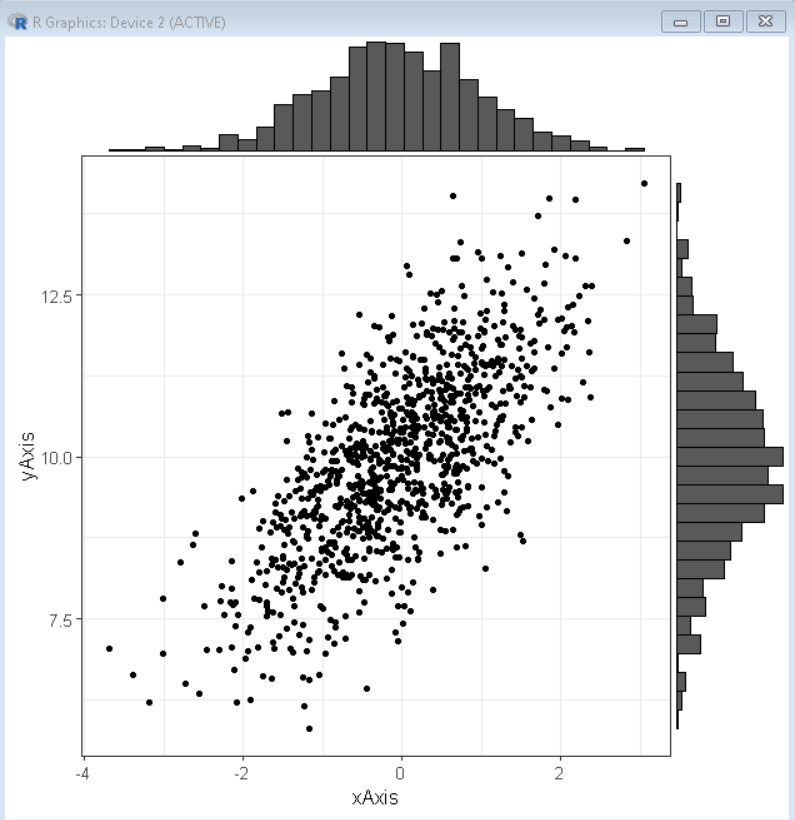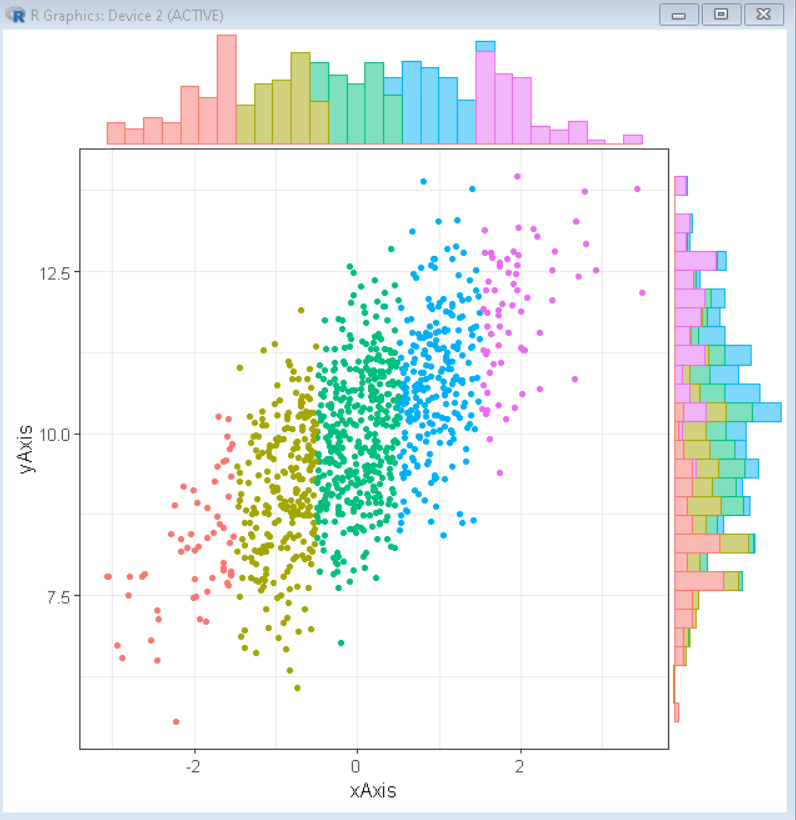Open in App
Not now

# How To Make Scatterplot with Marginal Histograms in R?

• Last Updated : 22 Nov, 2021

In this article, we will discuss how to make a Scatterplot with Marginal Histograms in the R Language.

To do so we will use the ggExtra package of the R Language. The ggExtra is a collection of functions and layers to enhance ggplot2. The ggMarginal() function can be used to add marginal histograms/boxplots/density plots to ggplot2 scatterplots.

### Installation:

To install the ggExtra package we use:

`install.packages("ggExtra")`

After installation, we can load the package and use the following function to make a marginal histogram with a scatter plot.

Syntax: ggMarginal( plot, type=”histogram” )

## Creating basic scatter plot with marginal histogram:

Here, is a basic scatter plot with marginal histogram using the ggMarginal function of ggExtra package.

## R

 `# load library tidyverse and ggExtra``library``(tidyverse)``library``(ggExtra)`` ` `# set theme``theme_set``(``theme_bw``(12))`` ` `# create x and y vector``xAxis <- ``rnorm``(1000)                 ``yAxis <- ``rnorm``(1000) + xAxis + 10  `` ` `# create sample data frame``sample_data <- ``data.frame``(xAxis, yAxis) `` ` `# create scatter plot using ggplot() function``plot <- ``ggplot``(sample_data, ``aes``(x=xAxis, y=yAxis))+``          ``geom_point``()+``        ``theme``(legend.position=``"none"``)`` ` `# use ggMarginal function to create marginal histogram``ggMarginal``(plot, type=``"histogram"``)`

Output:Output

## Color scatter plot with marginal histogram by group:

To color scatter plot by the group we use the col parameter of ggplot() function. To color the marginal histogram by the group we use groupColour and groupfill as true.

Syntax: ggMarginal( plot, type=”histogram”, groupColour = TRUE, groupFill = TRUE )

Example: Here, we have a scatter plot with marginal histograms both colored by the group. We use boolean values for groupColor and groupFill according to formatting preference.

## R

 `# load library tidyverse and ggExtra``library``(tidyverse)``library``(ggExtra)`` ` `# set theme``theme_set``(``theme_bw``(12))`` ` `# create x and y vector``xAxis <- ``rnorm``(1000)                 ``yAxis <- ``rnorm``(1000) + xAxis + 10    `` ` `# create groups in variable using conditional statements``group <- ``rep``(1, 1000)              ``group[xAxis > -1.5] <- 2``group[xAxis > -0.5] <- 3``group[xAxis > 0.5] <- 4``group[xAxis > 1.5] <- 5`` ` `# create sample data frame``sample_data <- ``data.frame``(xAxis, yAxis, group) `` ` `# create scatter plot using ggplot() ``# function colored by group``plot <- ``ggplot``(sample_data, ``aes``(x=xAxis, y=yAxis,``                                ``col = ``as.factor``(group)))+``          ``geom_point``()+``        ``theme``(legend.position=``"none"``)`` ` `# use ggMarginal function to create marginal histogram``ggMarginal``(plot, type=``"histogram"``,``           ``groupColour = ``TRUE``, groupFill = ``TRUE` `)`

Output:Output

My Personal Notes arrow_drop_up